A NUMERICAL COUPLING SCHEME FOR NONLINEAR TIME-HISTORY ANALYSIS OF BUILDINGS ON A REGIONAL SCALE CONSIDERING SITE-CITY INTERACTION EFFECTS

# Xinzheng Lu1,*, Yuan Tian2, Gang Wang3, and Duruo Huang4

1Key Laboratory of Civil Engineering Safety and Durability of China Education Ministry, Department of Civil Engineering, Tsinghua University, Beijing, P.R. China, 100084

2Beijing Engineering Research Center of Steel and Concrete Composite Structures, Tsinghua University, Beijing 100084, China

3Department of Civil and Environmental Engineering, Hong Kong University of Science and Technology, Clear Water Bay, Kowloon, Hong Kong

4Department of Hydraulic Engineering, Tsinghua University, Beijing, P.R. China, 100084

Correspondence

Xinzheng Lu, Key Laboratory of Civil Engineering Safety and Durability of China Education Ministry, Department of Civil Engineering, Tsinghua University, Beijing, P.R. China, 100084.

E-mail: luxz@tsinghua.edu.cn

Seismic damage simulation of buildings on a regional scale is important for loss estimation and disaster

mitigation of cities. However, the interaction among densely distributed buildings in a city and the site, i.e., the ˇ°site-city interaction (SCI) effectsˇ±, is often neglected in most regional simulations. Yet, many studies have found that the SCI effects are very important in regional simulations containing a large number of tall buildings and underground structures. Therefore, this work proposed a numerical coupling scheme for nonlinear time-history analysis of buildings on a regional scale considering the SCI effects. In this study, multiple-degree-of-freedom (MDOF) models are used to represent different buildings above the ground, while an open source spectral element program, SPEED, is used for simulating wave propagation in underlying soil layers. The proposed numerical scheme is firstly validated through a shaking table test. Then, a detailed discussion on the SCI effects in a 3D basin is performed. Finally, a nonlinear time-history analysis of buildings on a regional scale is performed using the Tsinghua University campus in Beijing as a case study. The Tsinghua University campus case results show that the SCI effects will reduce the seismic responses of most buildings. However, some buildings will suffer much more severe damage when the SCI effects are considered, which may depend on the input motions, site characteristics and building configurations.

# KEYWORDS

Regional seismic damage simulation; site-city interaction effect; multiple-degree-of-freedom model; SPEED; time-history analysis

# 1. INTRODUCTION

Earthquakes will cause a large amount of economic losses and casualties [Guha-Sapir et al. 2010, Guha-Sapir et al. 2011, Zeng et al. 2016], while a major part of seismic losses are contributed by building damages under earthquakes. Therefore, an accurate prediction of the seismic damage to buildings on a regional scale is important for city planning and post-earthquake rescue, which will help mitigate direct/indirect seismic losses [Hori 2006, FEMA 2012, Lu et al. 2014, FEMA P-366 2017].

Existing approaches for seismic damage evaluation of buildings on a regional scale mainly include: the damage probability matrix method [ATC-13 1985, Yakut et al. 2006], the capacity spectrum method [FEMA 2012], and methods based on time-history analysis (THA) [Hori 2006, Lu et al. 2017a]. The damage probability matrix method relies greatly on historical damage data, which is not easily adopted for areas without sufficient statistical data. The capacity spectrum method can, to some extent, consider the seismic resistance of individual buildings and the spectral characteristics of different ground motions. However, the effects of duration and pulse-like ground motions, or high-order vibration modes of buildings, cannot be fully considered by this method. Nowadays, the THA-based method has been widely used in regional seismic simulation with advancement in computer technology [Hori et al. 2008, Lu et al. 2017a]. The THA-based method can take full consideration of the nonlinear dynamic characteristics of buildings as well as intensity, frequency content and duration characteristics of ground motions, so it is particularly suitable for seismic damage simulation of buildings [Lu et al. 2014, Lu et al. 2017b]. To balance the accuracy and efficiency of the THA-based regional scale simulation containing a large number of buildings, Lu et al. [2017a] proposed a nonlinear multiple-degree-of-freedom (MDOF) shear model and a nonlinear MDOF flexural-shear model for different types of buildings. The accuracy and efficiency of these nonlinear MDOF models have been systematically validated by comparing the simulation results with a number of experimental results using actual earthquake records [Xiong et al. 2016, Xiong et al. 2017].

It is worth noting that, to date, the THA of buildings on a regional scale contains the following two steps: (1) the free-field ground motion is predicted by wave propagation modeling [Wang et al. 2018], stochastic simulation [Huang et al. 2015, Huang et al. 2017], or by using ground-motion prediction equations (GMPEs); (2) the THA of buildings is performed to predict the seismic damage under the predicted free-field ground motions. In this way, the site effects (SE) might be well considered, but the soil-structure interaction (SSI) and the structure-soil-structure interaction (SSSI) would be neglected. Note that SSI and SSSI would change the motions around buildings (The so-called ˇ°Contamination of Ground Motions by Buildingsˇ±, CGMB). Particularly, for densely populated cities, a large amount of multi-story and tall buildings are constructed closely to each other, which may greatly change the characteristics of sites. Such overall effects and the ˇ°globalˇ± interaction between all the buildings in a city and its subsoil are usually called ˇ°site-city interaction (SCI) effectsˇ± [Bard et al. 2006]. Bard et al.  found that, ˇ°if SCI effects turn out to be significant, one immediate consequence is that erecting or destroying a building, or a group of buildings, could modify seismic hazard for the neighbourhood, which in turn could lead to significant conceptual changes, especially concerning microzoning studies, land-use planning, and insurance policiesˇ±. Recently, many theoretical, experimental, and numerical studies have been performed on the SCI effects [Hori et al. 2008, Bard et al. 2006, Abraham et al. 2016, Boutin et al. 2014, Ghergu et al. 2009, Groby et al. 2008, Hans et al. 2005, Isbiliroglu et al. 2015, Kato et al. 2017, Kham et al. 2006, Lou et al. 2011, Mazzieri et al. 2013, Sahar et al. 2015, Sahar et al. 2016, Schwan et al. 2016, Semblat et al. 2008, Tsogka et al. 2003, Uenishi 2010]. For example, Schwan et al.  reported a series of shaking table tests, and found that densely located buildings can greatly change the characteristics of the site and the dynamic responses of buildings; Semblat et al.  performed the seismic wave propagation in an actual 2D shallow alluvial basin in Nice using Boundary Element Method, and analyzed the influence of SCI effects; Isbiliroglu et al.  analyzed the SCI effects of the Northridge Earthquake by using DRM (Domain Reduction Method) based on the Hercules system proposed by Tu et al. . Mazzieri et al.  developed an open source program SPEED (SPectral Elements in Elastodynamics with Discontinuous Galerkin) using spectral element method, and performed the seismic simulation considering SCI effects for Christchurch, New Zealand [Guidotti et al. 2012]. The program is also used in a recent case study of SCI effects for an urban transportation hub in Hong Kong [Kato et al. 2017].

Many existing studies proved that the ground motions can be affected by the existence of buildings, which may lead to a significant difference in the dynamic responses of buildings. For example, Isbiliroglu et al.  found that the vibration of large-scale structures will greatly affect the nearby buildings; Guidotti et al.  found that the SCI effects will change the ground motions by more than 1/3 compared with the free-field motions. Thus, the SCI effects should be well considered when conducting the seismic simulation of buildings on a regional scale.

Existing researches on SCI effects mainly focused on the wave propagation simulation. Instead, only very simple building models are adopted, such as elastic blocks, which are inaccurate and cannot consider the nonlinear behavior of real structures, and will lead to quite different simulation results from real structures. Therefore, based on existing studies, this work proposed a numerical coupling scheme for nonlinear time-history analysis of buildings on a regional scale considering the SCI effects. MDOF models, which can accurately simulate the nonlinear dynamic behaviors of buildings, are used to represent the key features of different buildings above the ground. An open source spectral element program, SPEED, is used to compute the wave propagation in underlying soil layers. The proposed numerical scheme is firstly validated through a shaking table test. Then, a detailed discussion on the SCI effects in a 3D basin is performed. Finally, a nonlinear THA of buildings on a regional scale is performed using the Tsinghua University campus in Beijing as a case study. The SCI effects to the seismic damage of buildings and the feasibility of the proposed method are discussed.

# 2. REGIONAL SCALE NONLINEAR THA OF BUILDINGS CONSIDERING SCI EFFECTS

## 2.1 Nonlinear MDOF model for buildings above the ground

Lu et al. [2017a] proposed that the nonlinear MDOF models can simulate the dynamic behavior of buildings with satisfactory accuracy and efficiency for regional seismic damage simulation. Therefore, the nonlinear MDOF models for buildings (including MDOF shear models and MDOF flexural-shear models as shown in Figures 1A and 1B) and the corresponding parameter determination method proposed by Lu et al. [2017a] and Xiong et al. [2016, 2017] are adopted in this work to perform the nonlinear THA of buildings on a regional scale.

In general, low- and multi-story buildings often exhibit shear deformation modes under earthquakes, while tall buildings will deform in flexure-shear modes. So the MDOF shear model will be used for the low- and multi-story buildings, and the MDOF flexural-shear model will be adopted to tall buildings. The masses of the buildings are concentrated on their corresponding stories, and the nonlinear behavior of the structure is represented by the nonlinear inter-story force-displacement relationships. Thus, the parameter determination of the inter-story force-displacement relationships will be very important for the rationality and accuracy of the simulation results, considering the limited available information for buildings on a regional scale.

Xiong et al. [2016, 2017] proposed a parameter determination and damage state determination method for the MDOF shear and flexural-shear models. In their work, trilinear backbone curves are adopted for the inter-story force-displacement relationships (shown in Figure 1C), and a single parameter pinching model proposed by Steelman et al.  is adopted. Firstly, based on building inventory data (including height, area, story number and so forth), a simulated design procedure is conducted according to the corresponding design codes, and the fundamental period and the design point on the backbone curve can be obtained. Then, according to related statistics of extensive experimental and analytical results, the yield point, peak point and softening point on the backbone curve can be further obtained, which in turn will determine the shape of the backbone curve. Five damage states, ranging from none, slight, moderate, to extensive and complete damage, are considered in their work. The reliability of the proposed method is further validated by comparing simulation results with actual seismic response.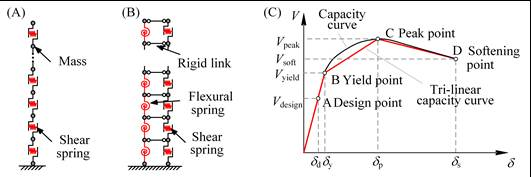Figure 1        (A) MDOF shear model; (B) MDOF flexural-shear model; and (C) Trilinear backbone curve adopted in MDOF model [Xiong et al. 2016, Xiong et al. 2017].

## 2.2 SPEED for simulating the wave propagation in 3D site models

To conveniently simulate the SCI effects, an open source program, SPEED [Mazzieri et al. 2013], is adopted in this work. The program can simulate seismic wave propagation in three-dimensional visco-elastic heterogeneous media on both local and regional scales. Based on the discontinuous Galerkin spectral approximation, SPEED can handle non-matching grids in an efficient and versatile way, and has been successfully applied in many cases, such as Christchurch in New Zealand, Thessaloniki in Greece and so forth [Abraham et al. 2016, Mazzieri et al. 2013, Evangelista et al. 2017, Smerzini et al. 2017].

The governing equation adopted in SPEED has the following expression to describe the wave propagation in the soil domain [Mazzieri et al. 2013]:(1)

where ¦Ń is the density of the soil; u,, and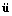represent the displacement, velocity and acceleration filed of the soil, respectively; ¦Î is the decay factor; ¦Ň(u) is the Cauchy stress tensor; and f is the density of body forces. The explicit Newmark method (¦Â = 0, ¦Ă = 0.5) is adopted for time integration in the dynamic simulation.

## 2.3 Numerical coupling scheme for simulating SCI effects

Figure 2 illustrates the numerical coupling scheme for simulating SCI effects. The numerical scheme consists of two main parts. In the first part, wave propagation in the soil domain is solved by using SPEED via Equation (1). In the second part, each building is modeled using the nonlinear MDOF model. To couple these two parts, in each time step, the base reaction forces from buildings are imposed to the soil domain, and the acceleration computed from the soil domain are set as base acceleration to buildings. The detailed computation procedure is illustrated in Figure 2 and shown as follows.

(a) Given the soil response and boundary condition at tn, the soil response at tn+1 ,, can be solved via Equation (1) by using explicit Newmark integration scheme (¦Â = 0, ¦Ă = 0.5). The equation can be written in a matrix form for the whole soil domain as follows: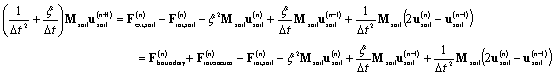(2)

where the superscript represents the time step; the external load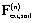applied to the soil domain at tn consists of two different sources: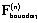represents boundary forces for seismic wave input at the bottom and wave absorption at the sides of truncated soil domain, and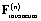is the interaction force imposed to the soil domain at each building location, which can be easily calculated as the base reaction force of the building from dynamic structural analysis;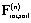denotes the internal load obtained from the soil response at tn; Msoil is the mass matrix for the soil domain. Note that Equation (2) has considered not only the soil-structure interaction at the building location, but also propagation and interaction of wave field due to inconsistency of soil motions at various locations, such that wave interaction among different building locations, and wave interaction between the near field and the free field can be naturally captured. It is also worth mentioning that heterogeneous soil layers and complex 3D topography can also be modeled using the spectral element simulation [Wang et al. 2018].

(b) After obtaining the soil displacement field at tn+1, the soil acceleration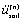at each building location (e.g., A and B in Figure 2) at tn can be assigned as base acceleration to each building using explicit Newmark method as shown in Equation (3).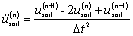(3)

(c) In this work, dynamic response analysis is performed individually for each building using the nonlinear MDOF model shown in Figure 1. The nonlinear structural responses of each building at tn+1,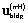, can be obtained by solving Equation (4):(4)

where {1} stands for a vector of ones {1, 1, ˇ­ , 1}T; Mbldg denotes the mass matrix of each building; Cbldg represents matrix for Rayleigh damping; Fint,bldg denotes the internal force obtained from the nonlinear building analysis. It is noted that in solving the nonlinear structural responses, the base of each building (e.g., A and B in Figure 2) is assumed to be fixed, and the base accelerationis imposed as inertia force to each story. Hence, the term ubldg in Figure (2) and Equation (4) represents the buildingˇŻs displacement relative to its base. As a result, the displacement compatibility at the building location is implicitly satisfied.

(d) Taken the base reaction force from each building at tn+1 as the updated interaction force, the updated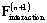is then applied to the soil domain at the building location for the next iteration.

(e) Loop over steps (a) to (d) until the last time step.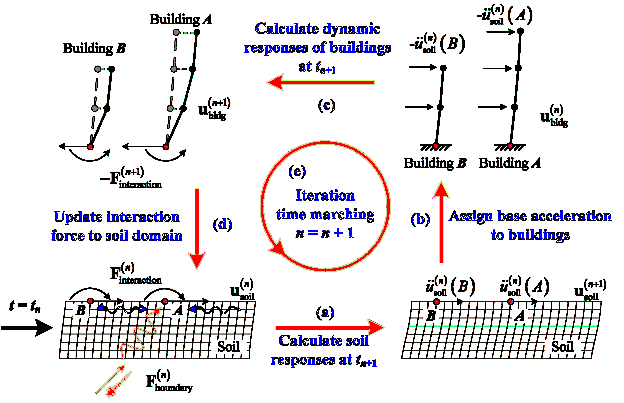Figure 2        Numerical coupling scheme for SCI effects.

To implement the above procedure, first of all, the building inventory data are necessary. The building inventory data include the height, story number, structural type, year built, location of the building, and other design information. The vibration period of the building is optional. If this information is not provided, the vibration period of the building will be estimated based on empirical equations and the building inventory data. Secondly, to update the interaction force Finteraction to the soil domain, the corresponding Neumann boundary conditions should be assigned according to the location of each building. A new function type for boundary conditions has been developed in SPEED, so that the boundary force can be updated at each time step by using the value of Finteraction calculated from the MDOF models.

Compared with existing models that simulate the SCI effects, the numerical coupling scheme proposed in this work requires only the building inventory data and the updated force boundary as additional input. The backbone curves of the buildings can be calculated automatically within the program according to Xiong et al.ˇŻs work [Xiong et al. 2016, Xiong et al. 2017], which significantly reduces the workload of numerical modeling. The MDOF model can capture the nonlinear seismic response of buildings, which cannot be achieved if an elastic block model is used [Kato and Wang 2017]. Owing to the reduced DOFs, the adoption of MDOF models for buildings is highly cost-effective for the simulation. The proposed method can not only consider the SSI at the building locations due to the coupling scheme, but also the SSSI and SCI effects, since they have been naturally captured during the dynamic soil response simulation in SPEED.

# 3. SHAKING TABLE TEST VALIDATION

A shaking table test [Schwan et al. 2016] is simulated to validate the proposed numerical coupling scheme. The prototype of the shaking table test is shown in Figure 3A. A cellular polyurethane foam, with a size of 2.13 m ˇÁ 1.76 m ˇÁ 0.76 m (X ˇÁ Y ˇÁ Z), is used to simulate the underlying foundation. The polyurethane foam has a density of 49 kg/m3, damping ratio of 4.9%, shear velocity of 33 m/s, and PoissonˇŻs ratio of 0.06. The fundamental frequency of the site in the X-direction is approximately 9.36 Hz according to the experimental measurement. Aluminum sheets with a height of 0.184 m and thickness of 0.5 mm are used to simulate multiple rows of buildings. The fundamental frequency of the aluminum sheets in the X-direction is approximately 8.45 Hz, with a damping ratio of approximately 4% according to the experimental results. The Ricker wavelet with spectral acceleration peak around 8 Hz is adopted as input signal to the bottom of the site. Two configurations, one having a single building, and the other having 37 buildings, are considered in the shaking table tests, as shown in Figure 3B.

To simulate this test, the site and the buildings are modeled separately. During the modeling procedure, the abovementioned parameters are adopted. The MDOF model with the same fundamental frequency, height, and mass is established, while the 3D solid model is adopted to simulate the site. The mesh used for the site model in the X-Z plane is shown in Figure 4. A mesh size of 93.75 mm is adopted along the Z-direction, and the mesh along the X-direction is determined according to the locations of the buildings. The first layer is established with a depth of 10 mm, so that the red part in Figure 4 can take the mass of the aluminum ˇ°foundationsˇ± (the aluminum angles at the bottom of the sheets) into consideration. The mesh size along the Y-direction is 0.05 m. A polynomial degree of 2 is adopted for the site model. Considering the shear velocity of the site (33 m/s) and the frequency range considered in this simulation (5¨C20 Hz), the average number of points per minimum wavelength is greater than 5; this shows that the mesh size adopted in this work can be considered to be reasonable [Komatitsch et al. 1999]. Based on the established model, the Ricker wavelet is adopted as the Dirichlet boundary condition at the bottom surface of the site model. During computation, the ground motions recorded at Point 1, and the corresponding transfer functions with respect to the input motion at the bottom of the site are compared, as shown in Figures 5 and 6.

Figures 5 and 6 show that the SCI effects will become more significant when the density of buildings is larger. The characteristics of the site can hardly be changed by only one building. But when the number of buildings is large enough, the SCI effects will lead to a smaller fundamental frequency of the site. In addition, two modes appear in the transfer function with smaller amplitudes (Figure 6B) instead of only one large peak (Figure 6A). The comparison between the simulation and test results demonstrate that the numerical coupling scheme proposed in this study can rather accurately simulate the influence of the SCI effects.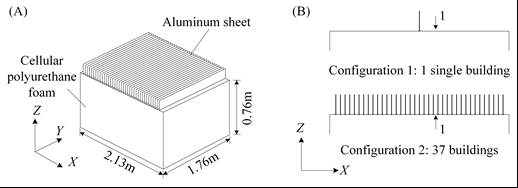Figure 3        (A) The prototype of the shaking table test; and (B) The configuration of buildings.Figure 4        Meshing scheme of the site model.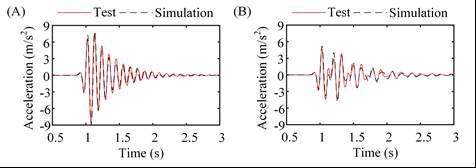Figure 5        Comparison of the ground motions at Point 1 under Configuration 1 (A) and Configuration 2 (B)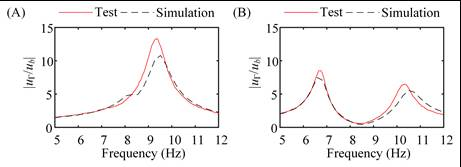Figure 6        Comparison of the transfer function |u¦Ł/ub| under Configuration 1 (A) and Configuration 2 (B).

# 4. CASE STUDY OF SCI EFFECTS IN A 3D BASIN

## 4.1 The model setup

Due to focusing effects, earthquake ground motions are often greatly amplified within a basin. In addition, double-resonance may occur if the fundamental period of a basin is close to that of the structure. Under double-resonance conditions, the SCI effects will be very significant according to previous studies [Kham et al. 2006, Semblat et al. 2008]. In this section, the method proposed in Section 2 will be adopted to perform a case study on the SCI effects in a 3D basin. To make full use of existing research outcomes, the trapezoidal (TRP) basin model analyzed by Sahar et al.  is adopted in this study. The buildings are considered to be elastic and the Ricker wavelet is used in the simulation. Note that, when detailed building inventory data are available, the numerical coupling scheme proposed in this study can simulate the nonlinear dynamic behavior of buildings, which will be shown in Section 5.

In this case, the size of the site considered is 3 km ˇÁ 3 km with a depth of 600 m. A TRP basin with a maximum depth of 150 m is located at the center of the site. The slope of the inclined base of the TRP basin is 30ˇă, and the detailed dimensions can be found in Figure 7. The rest of the site is made of rock, while the bottom layer with a depth of 100 m is used for plane wave input. The parameter of the site media is shown in Table 1. On the center of the basin surface, 9 building groups (denoted as B1 to B9) are arranged as 3 ˇÁ 3, while the centers of each building group on the surface are denoted as P1 to P9 as shown in Figure 8. The distance between neighboring building groups is 52 m. Each building group contains 9 buildings arranged as 3 ˇÁ 3 with a distance of 28m. The width and length of each building is 56 m, while the story height of each building is 3 m. The number of stories for these buildings vary in this case study.

The fundamental frequency of the basin is approximately 0.6 Hz according to the soil parameters of the basin. Thus, the Ricker wavelet with a dominant frequency of 0.6 Hz is adopted as the input motion along the X-axis. To achieve the double-resonance condition, 16-story buildings (with a fundamental frequency of 0.625 Hz) are placed on the ground surface of the basin. For comparison, buildings in several building groups are replaced by 8-story buildings, while the building locations are still the same, so that the influence of the height/frequency of the buildings on the SCI effect can be well simulated.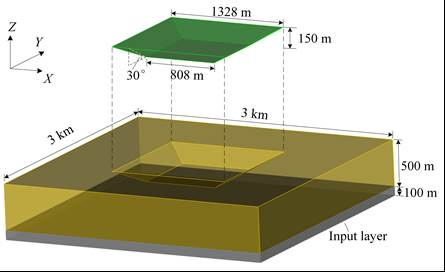Figure 7        The dimension of the TRP Basin model.Figure 8        The building configuration.

Table 1 Parameters of the basin and rock [Sahar et al. 2016]

 Material Density (kg/m3) VS (m/s) VP (m/s) QS* QP Basin 1800 360 612 36 61 Rock 2650 1800 3060 180 300

*The Q factor determines the qualitative behavior of damped oscillators. It equals 1/(2z), where z is the damping ratio [Thomson 1996].

Three cases are simulated and discussed in this study:

Case 1: The seismic wave propagation in the site is simulated under free field condition. And the building responses are simulated based on the free field motions;

Case 2: The SCI effects are considered during the computation, while all the buildings are 16-story buildings (denoted as Configuration A). This case is also a double-resonance condition;

Case 3: The SCI effects are also considered during the computation, while the buildings in the B2, B4, B6, and B8 groups are replaced by 8-story buildings (denoted as Configuration B).

The motions on the basin surface are recorded, and the ground motions at P1 to P9 will be analyzed in detail.

## 4.2 Case 1: building response under free-field motion without SCI effects

The peak ground acceleration and velocity (PGA and PGV) on the surface of the basin under Ricker wavelet are shown in Figures 9 and 10. Case 1 simulated the free field situation, in which no building is on the surface of the basin. However, the locations of buildings are also marked by white lines for the convenience of discussions in the following sections. Figures 9 and 10 show that, under the free field condition, although the Ricker wavelet is input along the X-axis, there are also ground motions along the Y-axis due to the reflection at the basin boundaries. At the same time, because of the symmetry of the site, the distribution of PGA and PGV are also symmetrical. In general, the center of the basin has the highest level of PGA and PGV. In addition, there are three peaks of the intensity (PGA and PGV) distribution along the Y-axis, with a distance of approximately 192 m. Because of the similar pattern of PGA and PGV, following sections will only discuss the differences of PGA distribution among the three cases in detail.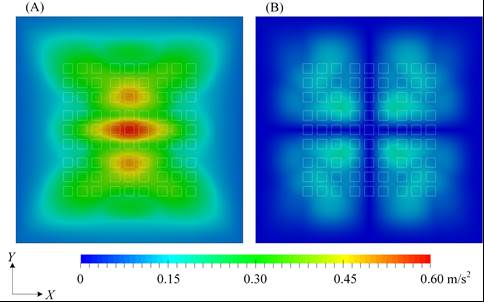Figure 9        Distribution of PGA under the free field condition (Case 1) along the X- (A), and Y-axis (B).Figure 10      Distribution of PGV under the free field condition (Case 1) along the X- (A), and Y-axis (B).

The transfer functions of the ground motions at P1 to P9 with respect to the input motion can be further obtained from the simulation. Due to the symmetry of the results, the transfer functions at (P1, P3, P7 and P9), (P2 and P8) and (P4 and P6) are also the same (Results of Case 2 and Case 3 in the following section also have the same conclusions). Therefore, only the transfer functions at P1, P2, P4, and P5 will be discussed. The transfer functions and response spectrum of ground motions at P1, P2, P4, and P5 are shown in Figure 11. According to Figure 11, the fundamental period of the basin is approximately 0.66 Hz, but different ground motions at different locations show different characteristics. In general, peak transfer functions are located in four frequency bands around 0.66Hz, 0.78-0.80 Hz, 1.00-1.07 Hz and 1.29-1.39 Hz.

Existing THA of buildings on a regional scale directly input the free field motions to the buildings for THA. Thus, both of the buildings in Configurations A and B are analyzed by inputting the free field motions obtained at their locations. The maximum roof drift ratio (RDR) of each building can be obtained as shown in Figure 12. For Configuration A, all of the 81 buildings have the same properties, so their responses are approximately proportional to the intensity (PGA) of the input motions. By contrast, for Configuration B, buildings in B2, B4, B6, and B8 are replaced by 8-story buildings, which in turn show smaller responses.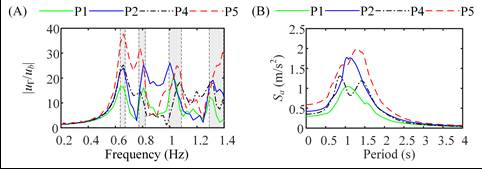Figure 11      Transfer function (A) and response spectrum (B) results at P1, P2, P4, and P5 in Case 1.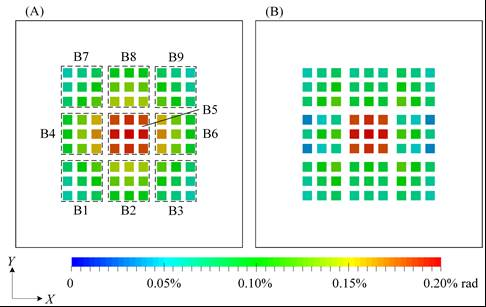Figure 12      The maximum RDR along the X-axis of buildings in Configuration A (A) and B (B) under free field ground motions (Case 1).

## 4.3 Case 2 and Case 3: building response considering SCI effects

Based on the numerical coupling scheme proposed in Section 2, the SCI effects are simulated in Case 2 and Case 3. Note that the PGV results have a very similar pattern with PGA results, so only PGA results will be discussed. The distribution of PGA on the surface of TRP basin under Ricker wavelet (with a dominant frequency of 0.6 Hz) is shown in Figure 13. The same legends are adopted as those in Figure 9, so that the influence caused by SCI effects can be illustrated easily. Since the ground motions are mainly along the X-axis, the results along the Y-axis will not be discussed. Compared with the results under free field condition, the SCI effects will greatly reduce the surface motion intensity under the double-resonance condition, which agrees with previous studies [Sahar et al. 2015, Semblat et al. 2008].

The increase ratios of PGA with respect to the results in Case 1 (free field condition) can be obtained, and are further shown in Figure 14 (Negative values mean reduction). The intensity of ground motions on the surface of the basin is generally reduced by the SCI effects with a maximum reduction of 24.98%. Further study shows that, the SCI effects can be very complex. For Case 2, all of 81 buildings on the surface of the basin are 16-story buildings. The reduction region of PGA has an elliptical shape, and the maximum reduction is located at the center of the ellipse, while some fluctuation exists in local areas. In addition, due to the reflection from the boundary of the basin and the wave radiated from buildings, the regions near building blocks along the Y-axis (denoted as R1 and R2 in Figure 14A) also have some intensity reductions. In terms of Case 3, buildings in B2, B4, B6, and B8 are replaced by 8-story buildings, which results in more complex results. The locations of B1 to B9 has been illustrated in Figure 14A. The PGA in B2 and B8 are hardly influenced by the SCI effects, with a reduction ratio of less than 10%. But in B4 and B6, more reduction in ground motion intensity is caused than those of Case 2. Similar to the results in Case 2, there are also some regions near the building blocks along the Y-axis where the intensity is greatly reduced. But Case 3 has more reductions than Case 2. Comparing results between Case 2 and Case 3, it can be found that the ground motions can also be influenced by the height/frequency of buildings, though the building density is the same.Figure 13      PGA distribution in Case 2 (A) and Case 3 (B) along the X-axis considering SCI effects.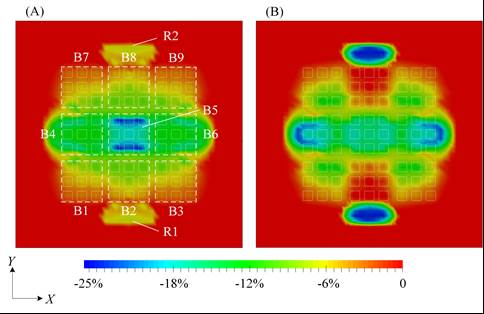Figure 14      Increase ratio of PGA on the surface of the basin in Case 2 (A) and Case 3 (B) compared with Case 1 (Negative value means reduction).

Figure 15 shows the increase ratio of maximum RDR of buildings in Case 2 and Case 3 considering SCI effects compared with Case 1. Compared with the results in Figure 14, it can be found that the building responses are influenced by the SCI effects in a similar way with the intensity of ground motions (PGA). In Case 2, the maximum reduction of RDR can be larger than 25% (25.91%); while in Case 3, the maximum reduction of RDR is less than 20% (18.82%). In general, the responses of buildings located at the middle row along X-axis will be influenced most significantly by the SCI effects due to the shape of the TRP basin.

In addition, the characteristics of the TRP basin can be studied from the outputs at P1 to P9. Considering the symmetry of results, only the results of P1, P2, P4, and P5 will be discussed. The comparison of the response spectrum of the ground motions at these points and the comparison of their transfer functions with respect to the input motion are shown in Figures 16 and 17. Note that the fundamental frequencies of the 16-story and 8-story buildings are 0.625 Hz and 1.25 Hz respectively. For Case 2, the transfer function values near the fundamental frequency of buildings (0.625 Hz) are greatly reduced, with a reduction of peak frequency as well (due to the inertia effect of buildings on the surface of the basin). The transfer function values in higher frequency range will also be reduced a bit. For Case 3, because the buildings located at P2 and P4 are 8-story buildings, the corresponding transfer function values will be further reduced around the frequency of 1.25 Hz. In addition, the fundamental frequency of the basin is also reduced due to the inertia effect, with a smaller amplitude as well. But the reduction of transfer function values is less than those in Case 2.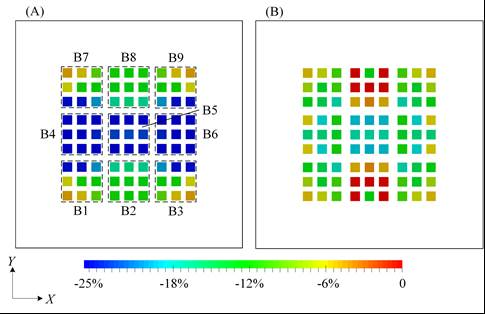Figure 15      Increase ratio of maximum RDR along the X-axis of buildings in Case 2 (A) and Case 3 (B) when considering SCI effects (Negative value means reduction).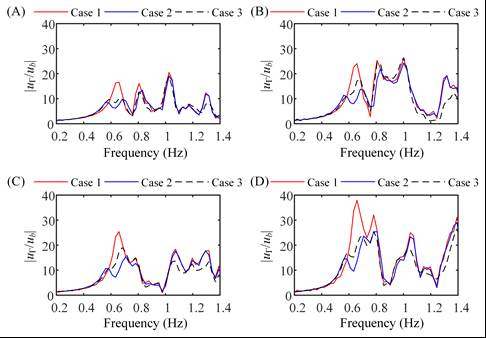Figure 16      Comparison of the transfer function results at P1 (A), P2 (B), P4 (C), and P5 (D) with/without SCI effects.

Figure 17 shows that the response spectrum of ground motions on the surface of the basin is generally reduced by the SCI effects. But it is also worth noting that the response spectrum value of P2 in Case 3 at the frequency of 1.2 Hz is larger than that in Case 1 (free field condition), which also reminds us that the response of buildings can also become larger due to SCI effects considering the complicated dynamic features of buildings and sites in real world.

According to above discussion, it can be found that for the 3D TRP basin studied in this section:

(1) Under the double-resonance condition (Case 2), the responses of buildings and the site will be reduced, which has been found in previous studies [Sahar et al. 2015, Semblat et al. 2008]. The method proposed in this work can fully represent this phenomenon caused by SCI effects. As a result, traditional seismic damage simulation of buildings on a regional scale (the free field motions are inputted directly to buildings) is conservative. However, it should be noted that, different from conventional design procedures (a conservative design is acceptable), a conservative prediction will overestimate the damage of buildings, which may in turn influence the distribution of relief supplies, and further restrict the efficiency of post-earthquake rescue work. Thus, it is of great value to take consideration of SCI effects in the seismic damage simulation of buildings.

(2) The existence of buildings will reduce the fundamental frequency of the site; and the transfer function amplitudes at the fundamental frequencies of both the buildings and the site will be reduced.

(3) Wave propagation in a basin can be quite complicated, and the reflection at the boundary of the basin will greatly influence the ground motions at the ground surface. Even if the building density on the surface of the basin keeps the same, the change of the building height/frequency will also significantly influence the SCI effects. Thus, the building density alone is not sufficient for measurement of SCI effects.Figure 17      Comparison of the spectral acceleration results at P1 (A), P2 (B), P4 (C), and P5 (D) with/without SCI effects.

# 5. CASE STUDY OF TSINGHUA UNIVERSITY CAMPUS

## 5.1 Introduction of the campus model

The Tsinghua University campus at Beijing is used to study the influence of SCI effects on the seismic damage simulation of buildings on a regional scale. The size of the region considered is 3 km ˇÁ 3 km ˇÁ 350 m (length ˇÁ width ˇÁ depth). Based on geological investigations and literature, the soil condition of the site is defined as follows:

(1) The thickness of the Quaternary deposits is 100 m [Pan et al. 2006], where the soil density is 2000 kg/m3;

(2) The thickness of the Tertiary deposits is 100 m [Fu et al. 2012], with a density of 2350 kg/m3;

(3) The shear velocity, VS, varies linearly from 200 m/s on the ground surface to 300 m/s in the depth of 30 m [Xie et al. 2016], and 1000m/s, 1800m/s at the bottom of the Quaternary and Tertiary deposits respectively [Fu et al. 2012]. The shear velocity profile is shown in Figure 18A;

(4) The bottom layer of the site is made of rock with a density of 2700 kg/m3 and a shear velocity of 3400 m/s [Fu et al. 2012]. In the simulation, the bedrock is truncated at 150 m in thickness.

By inputting the Ricker wavelet (with a dominant frequency of 2 Hz) to the bottom of the site model, the transfer function of the site (free filed motions with respect to input motions at the bedrock) is shown in Figure 18B, where the wave amplification peaks up at 1.2 Hz, 2.5 Hz and 4.0 Hz.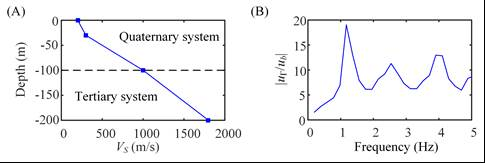Figure 18      (A) The distribution of the shear velocity; and (B) The transfer function of the site.

## 5.2 Seismic simulation of buildings considering SCI effects

Based on previous building survey data [Zeng et al. 2016], 619 buildings in Tsinghua University campus are considered. Corresponding MDOF models of buildings can be obtained according to the work by Xiong et al. [2016, 2017].

In this study, the Sanhe-Pinggu Earthquake (M8.0) scenario is considered, where Tsinghua University campus is located approximately 50 km to the earthquake epicenter. The target free-field ground motion is simulated and provided by the China Earthquake Administration [Fu et al. 2012] according to the scenario, as shown in Figure 19A. The SHAKE program [Schnabel et al. 1972] is used to perform the deconvolution of the ground motion to the bottom of the site, as shown in Figure 19B.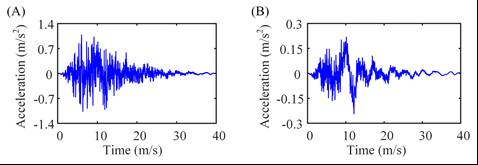Figure 19      (A) The free field ground motion; and (B) The deconvoluted motion at the bottom of the site.

Based on the site model and the input motion mentioned above, two cases are performed:

Case T1: The free filed motion is used directly as the input motion of the buildings, without considering the SCI effects;

Case T2: The proposed numerical coupling scheme is adopted to simulate wave propagation and interaction between the buildings and the site.

As demonstrated in Section 4, although Case T1 is usually adopted in seismic simulation of buildings on a regional scale, it does not consider the SCI effects, which may lead to different responses of buildings. So Case T2 is performed to study the influence of the SCI effects on the responses of buildings. Figure 20 shows the increase ratio of maximum RDR of buildings considering SCI effects and corresponding decomposition.Figure 20      (A) The distribution of increase ratio of maximum RDR; and (B) The composition of increase ratio of maximum RDR.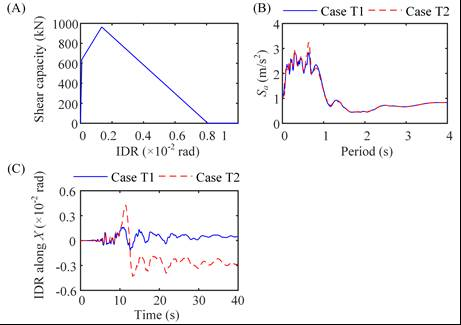Figure 21      (A) The backbone curve of the 1st floor in Building No. 559; (B) Comparison of response spectrum; and (C) Comparison of IDR responses of the 1st floor in Building No. 559.

Further study shows that, the consideration of the SCI effects will generally reduce the roof drift of buildings by 6.59% on average, while the maximum reduction can reach 45.72% and the maximum increase can be more than 50%. For most buildings, the increase/reduction ratio is no more than 25% (as shown in Figure 20B). However, 10 buildings, which are all unreinforced masonry buildings, suffer a large increase of roof drift for more than 50% due to low seismic resistances and ductility of these buildings, as small increase in ground motion will induce large increase in roof displacement responses. For example, Building No. 559 is a 3-story unreinforced building. The backbone curve for the 1st floor of the building is shown in Figure 21A. The response spectrum comparison of the motions at the structure location in the two cases is shown in Figure 21B. The inter-story drift ratio (IDR) responses of the 1st floor of Building No. 559 in Cases T1 and T2 are shown in Figure 21C. The difference between the motions in Figure 21B is far smaller than the responses in Figure 21C. This is because the inter-story shear-drift relationship of the building has reached the descending part of the backbone curve at approximately 10 s (the peak IDR of the backbone curve is 0.0013). At this moment, a small fluctuation of the input motion will collapse the first floor, and further lead to a much larger roof drift response.

From the distributions provided in Figure 20A, the mechanism of SCI effects can be very complex, due to the building distribution, the characteristics of the site and the input motions. From this case, the SCI effects will reduce the responses of most buildings. However, more severe damage occurs to several buildings. Such severe damage may lead to significant casualties. Therefore, the SCI effects should be well considered when performing nonlinear THA of buildings on a regional scale.

# 6. CONCLUSIONS

A numerical coupling scheme based on the MDOF models and the SPEED program is proposed in this work to simulate the SCI effect. The proposed method has been validated and successfully applied to the 3D basin site and Tsinghua University campus case study. Several conclusions can be drawn as follows:

(1) The validation of the shaking table test shows that the proposed numerical scheme can simulate the influence of the SCI effects with a satisfactory accuracy.

(2) Under the double-resonance condition, the responses of buildings and the site will be greatly reduced, which has been found in previous studies. The method proposed in this work can fully represent this characteristics caused by SCI effects.

(3) From the case study of the 3D basin, the building density alone is not sufficient for measuring the SCI effects. Some other factors, such as the building height, input motions, need to be considered together as well.

(4) In the case study of Tsinghua University campus, the SCI effects will generally reduce the structural responses. But some buildings may suffer more severe damage due to the SCI effects and the nonlinear behavior of buildings. Thus the SCI effects should be well considered during the nonlinear THA of buildings on a regional scale.

(5) The mechanism of SCI effects can be very complex, which will be greatly influenced by the building configuration, site characteristics, input motions and so forth. The numerical scheme proposed in this work can provide a useful simulation tool to researches on SCI effects, and more generalized conclusions will be discussed in the future.

# ACKNOWLEDGEMENT

The authors would like to acknowledge the financial supports of National Natural Science Foundation of China (No. 51578320), Hong Kong Research Grants Council (GRF 16214118) and HKUST Department of Civil and Environmental Engineering Intergroup Collaborative Research Program. The authors would like also to acknowledge Dr. Changhua Fu from China Earthquake Administration and Mr. Qingle Cheng for their contribution to this work.

# REFERENCE

Abraham JR, Smerzini C, Paolucci R, Lai CG. Numerical study on basin-edge effects in the seismic response of the Gubbio valley, Central Italy. Bulletin of Earthquake Engineering. 2016; 14(6):1437-1459.

ATC-13. Earthquake damage evaluation data for California. Redwood City, California: Applied Technology Council, 1985.

Bard PY, Chazelas J, Gu¨¦guen P, Kham M, Semblat JF. Site-City Interaction. In: Assessing and Managing Earthquake Risk. Dordrecht: Springer, 2006: 91-114.

Boutin C, Soubestre J, Schwan L and Dietz M. Multi-scale modeling for dynamics of structure-soil-structure interactions. Acta Geophysica. 2014; 62(5):1005-1024.

Evangelista L, del Gaudio S, Smerzini C, dˇŻOnofrio A, Festa G, Iarvolino I, Landolfi L, Paolucci R, Santo A, Silvestri F. Physics-based seismic input for engineering applications: a case study in the Aterno river valley, Central Italy. Bulletin of Earthquake Engineering. 2017; 15(7):2645-2671.

FEMA. Multi-hazard loss estimation methodology: earthquake model (HAZUS-MH 2.1 technical manual). Washington, DC: Federal Emergency Management Agency, 2012.

FEMA P-366. Hazus® estimated annualized earthquake losses for the United State. Washington, DC: Federal Emergency Management Agency, 2017.

Fu CH. A study on long-period acceleration response spectrum of ground motion affected by basin structure of Beijing. Dissertation, Institute of Geophysics, China Earthquake Administration, Beijing, China; 2012.

Ghergu M, Ionescu IR. Structure-soil-structure coupling in seismic excitation and ˇ°city effectˇ±. International Journal of Engineering Science. 2009; 47(3):342-354.

Groby JP, Wirgin A. Seismic motion in urban sites consisting of blocks in welded contact with a soft layer overlying a hard half-space. Geophysical Journal International. 2008; 172(2):725-758.

Guha-Sapir D, Vos F, Below R, Ponserre S. Annual disaster statistical review 2010: The numbers and trends. Centre for Research on the Epidemiology of Disasters (CRED). Universite´ catholique de Louvain, Brussels, Belgium, 2011.

Guha-Sapir D, Vos F, Below R and Ponserre S. Annual disaster statistical review 2011: The numbers and trends. Centre for Research on the Epidemiology of Disasters (CRED). Institute of Health and Society (IRSS), Universite´ catholique de Louvain, Brussels, Belgium, 2012

Guidotti R, Mazzieri I, Stupazzini M, Dagna P. 3D numerical simulation of the site-city interaction during the 22 February 2011 MW 6.2 Christchurch earthquake. In: 15th World Conference of Earthquake Engineering. Lisboa, Portugal, 2012.

Hans S, Boutin C, Ibraim E, Roussillon P. In situ experiments and seismic analysis of existing buildings. Part I: Experimental investigations. Earthquake Engineering & Structural Dynamics. 2005; 34(12):1513-1529.

Hori M. Introduction to computational earthquake engineering. London: Imperial College Press, 2006.

Hori M, Ichimura T. Current state of integrated earthquake simulation for earthquake hazard and disaster. Journal of Seismology. 2008; 12(2):307-321.

Huang D, Wang G. Stochastic Simulation of Regionalized Ground Motions using Wavelet Packet and Cokriging Analysis. Earthquake Engineering & Structural Dynamics. 2015; 44: 775-794.

Huang D, Wang G. Energy-compatible and spectrum-compatible (ECSC) ground motion simulation using wavelet packets. Earthquake Engineering & Structural Dynamics. 2017; 46:1855-1873.

Isbiliroglu Y, Taborda R, Bielak J. Coupled soil-structure interaction effects of building clusters during earthquakes. Earthquake Spectra. 2015; 31(1): 463-500.

Kato B, Wang G. Ground motion simulation in an urban environment considering site-city interaction: a case study of Kowloon station, Hong Kong. In: 3rd Huixian International Forum on Earthquake Engineering for Young Researchers. University of Illinois, Urbana-Champaign, United States, August 11-12, 2017.

Kham M, Semblat JF, Bard PY, Dangla P. Seismic site¨Ccity interaction: main governing phenomena through simplified numerical models. Bulletin of the Seismological Society of America. 2006; 96(5):1934-1951.

Komatitsch D, Tromp J. Introduction to the spectral element method for three-dimensional seismic wave propagation. Geophysical Journal International. 1999; 139(3):806-822.

Lou M, Wang H, Chen X, Zhai YM. Structure-soil-structure interaction: literature review. Soil Dynamics and Earthquake Engineering. 2011; 31(12):1724-1731.

Lu XZ, Han B, Hori M, Xiong C, Xu Z. A coarse-grained parallel approach for seismic damage simulations of urban areas based on refined models and GPU/CPU cooperative computing. Advances in Engineering Software. 2014; 70:90-103.

Lu XZ, Guan H. Earthquake disaster simulation of civil infrastructures: from tall buildings to urban areas. Singapore: Springer, 2017a.

Lu XZ, Tian Y, Guan H, Xiong C. Parametric sensitivity study on regional seismic damage prediction of reinforced masonry buildings based on time-history analysis. Bulletin of Earthquake Engineering. 2017b; 15(11):4791-4820.

Mazzieri I, Stupazzini M, Guidotti R, Smerzini C. SPEED: SPectral Elements in Elastodynamics with Discontinuous Galerkin: a non-conforming approach for 3D multi-scale problems. International Journal for Numerical Methods in Engineering. 2013; 95(12):991-1010.

Pan B, Xu JD, Haruko S, He HL. Simulation of the near-fault strong ground motion in Beijing region. Seismology and Geology. 2006; 28(4):623-634.

Sahar D, Narayan JP, Kumar N. Study of role of basin shape in the site¨Ccity interaction effects on the ground motion characteristics. Natural Hazards. 2015; 75(2):1167-1186.

Sahar D, Narayan JP. Quantification of modification of ground motion due to urbanization in a 3D basin using viscoelastic finite-difference modelling. Natural Hazards. 2016; 81(2):779-806.

Schnabel PB, Lysmer J, Seed HB. SHAKE: a computer program for earthquake response analysis of horizontal layered sites. In: Earthquake Engineering Research Center (EERC) Report, University of California at Berkeley, 1972.

Schwan L, Boutin C, Padr¨®n LA, Dietz MS, Bard PY, Taylor C. Site-city interaction: theoretical, numerical and experimental crossed-analysis. Geophysical Journal International. 2016; 205(2):1006-1031.

Semblat JF, Kham M, Bard PY. Seismic-wave propagation in alluvial basins and influence of site-city interaction. Bulletin of the Seismological Society of America. 2008; 98(6):2665-2678.

Smerzini C, Pitilakis K, Hashemi K. Evaluation of earthquake ground motion and site effects in the Thessaloniki urban area by 3D finite-fault numerical simulations. Bulletin of Earthquake Engineering. 2017; 15(3):787-812.

Steelman JS, Hajjar JF. Influence of inelastic seismic response modeling on regional loss estimation. Engineering Structures. 2009; 31(12):2976-2987.

Thomson W. Theory of vibration with applications. CRC Press, 1996.

Tsogka C, Wirgin A. Simulation of seismic response in an idealized city. Soil Dynamics and Earthquake Engineering. 2003; 23(5):391-402.

Tu T, Yu H, Ramirez-Guzman L, Bielak J, Ghattas O, Ma KL, OˇŻHallaron DR. From mesh generation to scientific visualization: An end-to-end approach to parallel supercomputing. In: Proceedings of the 2006 ACM/IEEE conference on Supercomputing, 2006.

Uenishi K. The town effect: dynamic interaction between a group of structures and waves in the ground. Rock Mechanics and Rock Engineering. 2010; 43(6):811-819.

Wang G, Du C, Huang D, Jin F, Koo RCH, Kwan JSH. Parametric models for ground motion amplification considering 3D topography and subsurface soils. Soil Dynamics and Earthquake Engineering. 2018; accepted.

Xie JJ, Zimmaro P, Li XJ, Wen ZP, Song YS. VS30 Empirical Prediction Relationships Based on a New Soil-Profile Database for the Beijing Plain Area, China. Bulletin of the Seismological Society of America. 2016; 106(6):2843-2854.

Xiong C, Lu XZ, Guan H and Xu Z. A nonlinear computational model for regional seismic simulation of tall buildings. Bulletin of Earthquake Engineering. 2016; 14(4):1047-1069.

Xiong C, Lu XZ, Lin XC, Xu Z and Ye LP. Parameter determination and damage assessment for THA-based regional seismic damage prediction of multi-story buildings. Journal of Earthquake Engineering. 2017; 21(3): 461-485.

Yakut A, Ozcebe G, Yucemen MS. Seismic vulnerability assessment using regional empirical data. Earthquake Engineering & Structural Dynamics. 2006; 35(10):1187-1202.

Zeng X, Lu XZ, Yang T, Xu Z. Application of the FEMA-P58 methodology for regional earthquake loss prediction. Natural Hazards. 2016; 83(1):177-192.